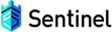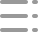En# Sentinel Go 毫秒级统计数据结构揭秘

## 固定窗口

``````var (
counter    int64 //计数
intervalMs int64 = 1000 //窗口长度(1S)
threshold  int64 = 2 //限流阈值
startTime        = time.Now().UnixMilli() //窗口开始时间
)

func main() {
for i := 0; i < 10; i++ {
if tryAcquire() {
fmt.Println("成功请求", time.Now().Unix())
}
}
}

func tryAcquire() bool {
atomic.StoreInt64(&startTime, time.Now().UnixMilli())
atomic.StoreInt64(&counter, 0)
}
}
``````## 滑动时间窗口

• 统计周期：例如想限制5S的请求数不能超过100次，那么5S就是统计周期
• 窗口(格子)的大小：一个周期内会有多个窗口(格子)进行指标（例如请求数）的统计，长度相等的统计周期，格子的数量越多，统计的越精确• 统计周期一致，窗口大小不一致：窗口越大统计精准度越低，但并发性能好；窗口越小，统计精准度越高，但并发性能随之降低
• 统计周期不一致，窗口大小一致：周期越长抗流量脉冲情况越好

## 统计结构

### 窗口结构

``````type BucketWrap struct {
// BucketStart represents start timestamp of this statistic bucket wrapper.
BucketStart uint64
// Value represents the actual data structure of the metrics (e.g. MetricBucket).
Value atomic.Value
}
``````
• 开始时间：当前格子的的起始时间
• 统计结构：存储指标数据，原子操作并发安全1. pass: 表示到来的数量，即此刻通过 Sentinel-Go 规则的流量数量
2. block: 表示被拦截的流量数量
3. complete: 表示完成的流量数量，包含正常结束和异常结束的情况
4. error: 表示错误的流量数量（熔断场景使用）
5. rt： 单次请求的request time
6. total：暂时无用

### 原子时间轮``````type AtomicBucketWrapArray struct {
// The base address for real data array
base unsafe.Pointer // 窗口数组首元素地址
// The length of slice(array), it can not be modified.
length int // 窗口数组的长度
data   []*BucketWrap //窗口数组
}
``````

#### 初始化

1. 根据当前时间计算出当前时间对应的窗口的startime，并得到当前窗口对应的位置：
``````// 计算开始时间
func calculateStartTime(now uint64, bucketLengthInMs uint32) uint64 {
return now - (now % uint64(bucketLengthInMs))
}
// 窗口下标位置
idx := int((now / uint64(bucketLengthInMs)) % uint64(len))
``````1. 初始化窗口数据结构（BucketWrap）
``````for i := idx; i <= len-1; i++ {
ww := &BucketWrap{
BucketStart: startTime,
Value:       atomic.Value{},
}
ww.Value.Store(generator.NewEmptyBucket())
ret.data[i] = ww
startTime += uint64(bucketLengthInMs)
}
for i := 0; i < idx; i++ {
ww := &BucketWrap{
BucketStart: startTime,
Value:       atomic.Value{},
}
ww.Value.Store(generator.NewEmptyBucket())
ret.data[i] = ww
startTime += uint64(bucketLengthInMs)
}
``````1. 将窗口数组首元素地址设置到原子时间轮：
``````// calculate base address for real data array
``````

#### 窗口获取&窗口替换

``````// 获取对应窗口
func (aa *AtomicBucketWrapArray) get(idx int) *BucketWrap {
// aa.elementOffset(idx) return the secondary pointer of BucketWrap, which is the pointer to the aa.data[idx]
// then convert to (*unsafe.Pointer)
if offset, ok := aa.elementOffset(idx); ok {
}
return nil
}

// 替换对应窗口
func (aa *AtomicBucketWrapArray) compareAndSet(idx int, except, update *BucketWrap) bool {
// aa.elementOffset(idx) return the secondary pointer of BucketWrap, which is the pointer to the aa.data[idx]
// then convert to (*unsafe.Pointer)
// update secondary pointer
if offset, ok := aa.elementOffset(idx); ok {
return atomic.CompareAndSwapPointer((*unsafe.Pointer)(offset), unsafe.Pointer(except), unsafe.Pointer(update))
}
return false
}

// 获取对应窗口的地址
func (aa *AtomicBucketWrapArray) elementOffset(idx int) (unsafe.Pointer, bool) {
if idx >= aa.length || idx < 0 {
logging.Error(errors.New("array index out of bounds"),
"array index out of bounds in AtomicBucketWrapArray.elementOffset()",
"idx", idx, "arrayLength", aa.length)
return nil, false
}
basePtr := aa.base
return unsafe.Pointer(uintptr(basePtr) + uintptr(idx)*unsafe.Sizeof(basePtr)), true
}
``````

1. 在get func中接收根据当前时间计算出的窗口对应下标位置
2. 根据下标位置在elementOffset func中，首先将底层的slice首元素地址转换成uintptr，然后将窗口对应下标*对应的指针字节大小即可以得到对应窗口元素的地址
3. 将对应窗口地址转换成时间窗口（`*BucketWarp`）即可### 滑动窗口

#### 滑动

``````// 根据当前时间获取周期内的所有窗口
func (m *SlidingWindowMetric) getSatisfiedBuckets(now uint64) []*BucketWrap {
start, end := m.getBucketStartRange(now)
satisfiedBuckets := m.real.ValuesConditional(now, func(ws uint64) bool {
return ws >= start && ws <= end
})
return satisfiedBuckets
}

// 根据当前时间获取整个周期对应的窗口的开始时间和结束时间
func (m *SlidingWindowMetric) getBucketStartRange(timeMs uint64) (start, end uint64) {
curBucketStartTime := calculateStartTime(timeMs, m.real.BucketLengthInMs())
end = curBucketStartTime
start = end - uint64(m.intervalInMs) + uint64(m.real.BucketLengthInMs())
return
}

// 匹配符合条件的窗口
func (la *LeapArray) ValuesConditional(now uint64, predicate base.TimePredicate) []*BucketWrap {
if now <= 0 {
return make([]*BucketWrap, 0)
}
ret := make([]*BucketWrap, 0, la.array.length)
for i := 0; i < la.array.length; i++ {
ww := la.array.get(i)
if ww == nil || la.isBucketDeprecated(now, ww) || !predicate(atomic.LoadUint64(&ww.BucketStart)) {
continue
}
ret = append(ret, ww)
}
return ret
}
````````````satisfiedBuckets := m.real.ValuesConditional(now, func(ws uint64) bool {
return ws >= start && ws <= end
})
``````

#### 更新``````func (la *LeapArray) currentBucketOfTime(now uint64, bg BucketGenerator) (*BucketWrap, error) {
// 计算当前时间对应的窗口下标
idx := la.calculateTimeIdx(now)
// 计算当前时间对应的窗口的开始时间
bucketStart := calculateStartTime(now, la.bucketLengthInMs)

for {
// 获取旧窗口
old := la.array.get(idx)
// 如果旧窗口==nil则初始化(正常不会执行这部分代码)
if old == nil {
newWrap := &BucketWrap{
BucketStart: bucketStart,
Value:       atomic.Value{},
}
newWrap.Value.Store(bg.NewEmptyBucket())
if la.array.compareAndSet(idx, nil, newWrap) {
return newWrap, nil
} else {
runtime.Gosched()
}
// 如果本次计算的开始时间等于旧窗口的开始时间，则认为窗口没有过期，直接返回
} else if bucketStart == atomic.LoadUint64(&old.BucketStart) {
return old, nil
//  如果本次计算的开始时间大于旧窗口的开始时间，则认为窗口过期尝试重置
} else if bucketStart > atomic.LoadUint64(&old.BucketStart) {
if la.updateLock.TryLock() {
old = bg.ResetBucketTo(old, bucketStart)
la.updateLock.Unlock()
return old, nil
} else {
runtime.Gosched()
}
......
}
}
``````

## 总结

Sentinel Go 的整体的数据结构图：